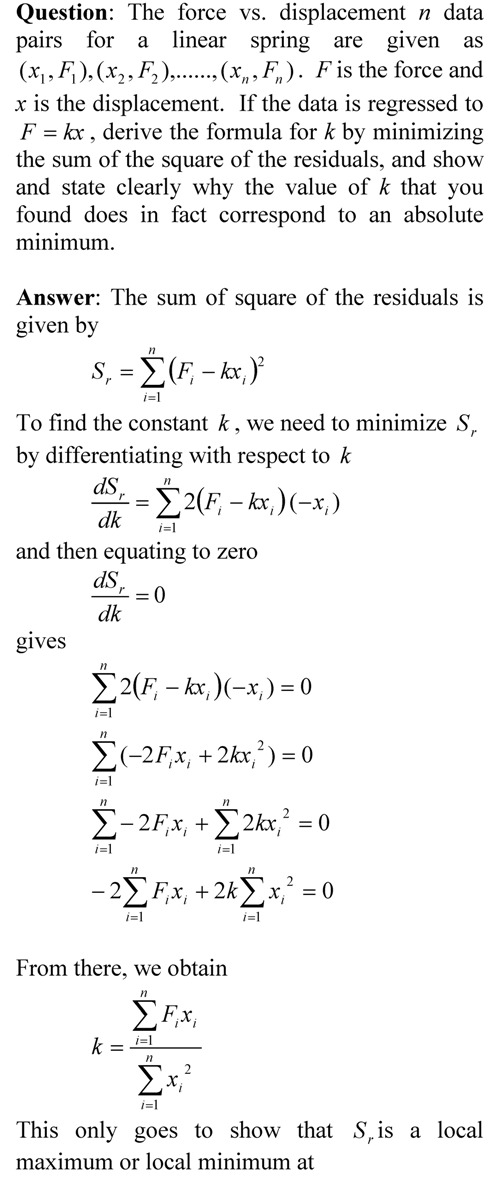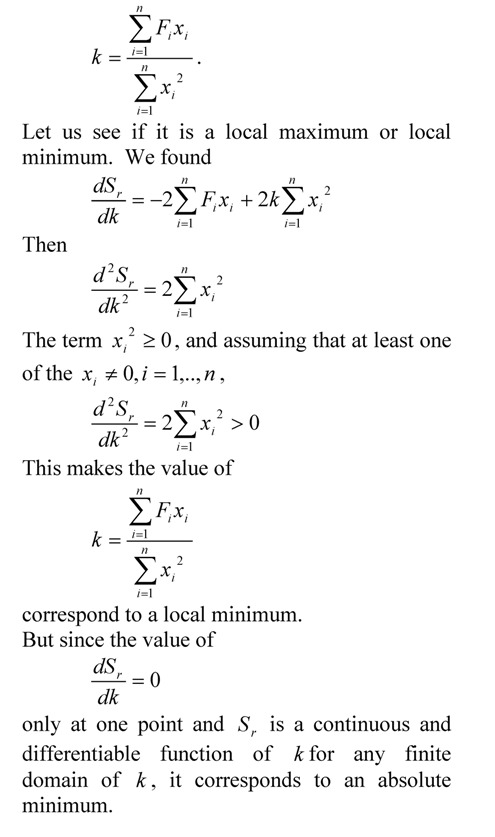# To prove that the regression model corresponds to a minimum of the sum of the square of the residuals

Many regression models when derived in books only show the first derivative test to find the formulas for the constants of a regression model.  Here we take a  simple example to go through the complete derivation._________________________________________________________

This post is brought to you by Holistic Numerical Methods: Numerical Methods for the STEM undergraduate at http://nm.mathforcollege.com, the textbook on Numerical Methods with Applications available from the lulu storefront, and the YouTube video lectures available at http://nm.mathforcollege.com/videos and http://www.youtube.com/numericalmethodsguy